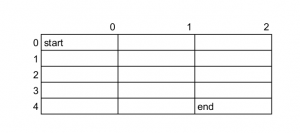# Maths behind number of paths in matrix problem

The problem is to count all the possible paths from top left to bottom right of an m*n matrix with the constraints that from each cell you can either move only towards right or down.

First of all read various possible solutions for the stated problem here

Now for the last solution, the combination formula for computing number of paths is given as m + n – 2Cm – 1. Let’s discuss the maths behind that formula.

Suppose we have an m*n matrix then according to the question we can only move right or down.Here m = 5 and n = 3, we start from (0, 0) (i.e. start) and go to the end i.e. (4, 2) we can consider any one path lets say we choose
(0, 0) -> (0, 1) -> (0, 2) -> (1, 2) -> (2, 2) -> (3, 2) -> (4, 2)
Therefore, we moved 2 steps to the right and 4 steps downwards. Even if we take any other path same number of right and down steps will be required.

Now recall the combination in maths. It is just that where instead of letters we have paths. Here we have to cover n-1 + m-1 cellular length to destination.
Also recall that we are moving m-1 steps in downward direction and n-1 in right direction. Therefore the number of paths will essentially be (m + n – 2)! / (n – 1)! * (m – 1)! which is nothing but m + n – 2Cn – 1 or m + n – 2Cm – 1.

My Personal Notes arrow_drop_upCheck out this Author's contributed articles.

If you like GeeksforGeeks and would like to contribute, you can also write an article using contribute.geeksforgeeks.org or mail your article to contribute@geeksforgeeks.org. See your article appearing on the GeeksforGeeks main page and help other Geeks.

Please Improve this article if you find anything incorrect by clicking on the "Improve Article" button below.